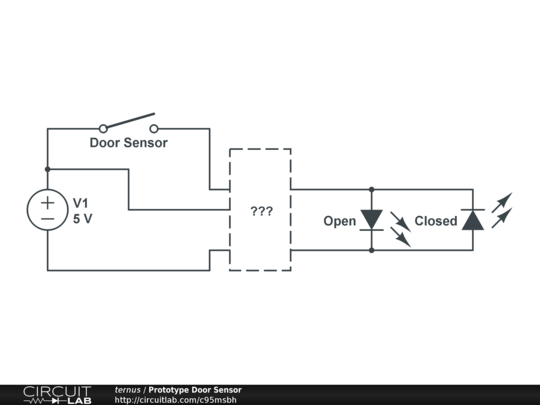# Control two LEDs with two wires and inverter

I have a door sensor (magnetic Hall Effect) that I want to use to build a simple indicator: red LED if closed, green LED if open.

Here's the tricky part: I want to run only two wires to those LEDs, and I'd like to do it completely in analog circuitry. Here's the approximate outline:I've tried putting something simple together with BJTs (an inverter, a bistable multivibrator etc.), and I feel like this is the right track -- that there's just one small tweak I need to make -- but I'm stumped as to how to get the voltage to flow. I've done extensive Googling and reading up on tutorials and I've found nothing close to what I'm looking for.

How do I get voltage to flow one direction if the switch is closed and in the other direction if it's open?

• Use a DPDT relay. Dec 5, 2013 at 2:10
• @ChristianTernus For future reference. You wrote initially "[...] the voltage to flow". Current flows. Voltage does not flow; however voltage (or voltage difference, to be more precise) causes the current to flow. Dec 5, 2013 at 2:29
• @ChristianTernus To give you a water analogy: electric current is like flow of water in the pipe, voltage is like pressure in the pipe. It's possible to have pressure without flow. It's possible to have a voltage without current. (While the water analogy is handy in the beginning, there are plenty of difference between electric current flow and liquid flow. If you think about it more, the analogy will break down. You can go only so far with it.) Dec 5, 2013 at 2:33
• @NickAlexeev Thanks -- I plead guilty of using imprecise terminology :) Substitute current for voltage, then. Dec 5, 2013 at 2:36
• Use two I/O pins on an MCU to drive the LED's and another I/O pin to sense the switch position. Dec 5, 2013 at 2:40

When using a red and green LED, you can utilize the difference in LED forward voltage as in the circuit below. Make sure to use high efficiency LED's. These have a low internal resistance and a nice and sharp bend in the forward voltage/current curve.simulate this circuit – Schematic created using CircuitLab

Normally the green LED lights, which has a forward voltage of approximately 2V. Then when the switch closes, the red LED light and due to its lower forward voltage, approx. 1.8V, the green LED will turn off from (voltage) starvation.

If the green LED does not entirely turn off, you can add a regular 1N4148 diode in series with it to make the effect stronger, but this is usually only necessary with cheap and relatively low efficiency LED's.

To keep it "analog", you could use two op-amps connected in a bridge-tied-load configuration, but configured as comparators.

When one comparator swings high, the other swings low and vice versa, i.e:simulate this circuit – Schematic created using CircuitLab

This is how you get the voltage to reverse. If the IN voltage is above VREF, then it goes one way. If IN is below VREF, it goes the other way. Achieving the IN movement between two voltages is trivial. For instance, IN can be connected to your positive voltage via a pull-up resistor, and the switch can be wired to ground IN when closed.

You could build this with discrete circuits, but op-amps have the gain for a sharp transition.

A possible refinement would be to give the circuit some hysteresis (Schmitt-trigger behavior), so that it swings positive above some higher VREF1, and swings negative below some VREF0 < VREF1.

I put in the LM358 part number, which is a good op-amp for this because it supports single supply-operation well: the inputs can go all the way to the negative supply rail, and below. So if the IN voltage goes to ground, that chip won't have a fit. The current sourcing and sinking ability is not that great; if you only need up to around 10 mA through the LEDs, it should be fine.

This might be helpful if you are looking for a pure analog solution without logic gates/relays or integrated circuits.simulate this circuit – Schematic created using CircuitLab

Disclaimer: This answer is purely from an academic perspective. Consider that it may be wrong and/or not safe for practice. Any harm caused from building and operating this circuit, including but not limited to electric shock, is the responsibility of the circuit assembler.

It's no different to using a chip to reverse a motor. It's called a H bridge. Here's one that should work: -You'd need an inverter to drive one of the inputs but other than that it emulates a DPDT relay.

I bet the idea below can be optimized further, but I don't have time right now to improve it. How about this proof of concept:simulate this circuit – Schematic created using CircuitLab

Not the most power efficient solution with the resistive divider, but cheap solution and requires only two leads to the LEDs.

Here is a solution based on a DPDT relay (long version of Ignacio's comment I guess :-)